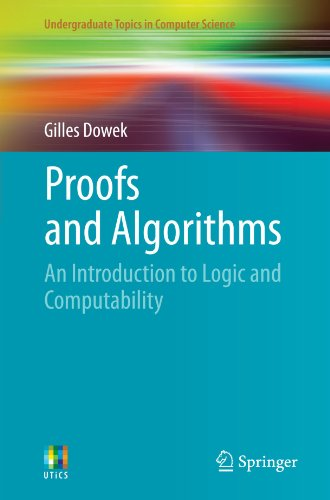# Proofs and Algorithms: An Introduction to Logic and Computability

• Length: 203 pages
• Edition: 1st Edition.
• Publisher:
• Publication Date: 2011-01-13
• ISBN-10: 0857291203
• ISBN-13: 9780857291202
4.0
Description

Logic is a branch of philosophy, mathematics and computer science. It studies the required methods to determine whether a statement is true, such as reasoning and computation. Proofs and Algorithms: Introduction to Logic and Computability is an introduction to the fundamental concepts of contemporary logic – those of a proof, a computable function, a model and a set. It presents a series of results, both positive and negative, – Church’s undecidability theorem, Gödel’s incompleteness theorem, the theorem asserting the semi-decidability of provability – that have profoundly changed our vision of reasoning, computation, and finally truth itself. Designed for undergraduate students, this book presents all that philosophers, mathematicians and computer scientists should know about logic.